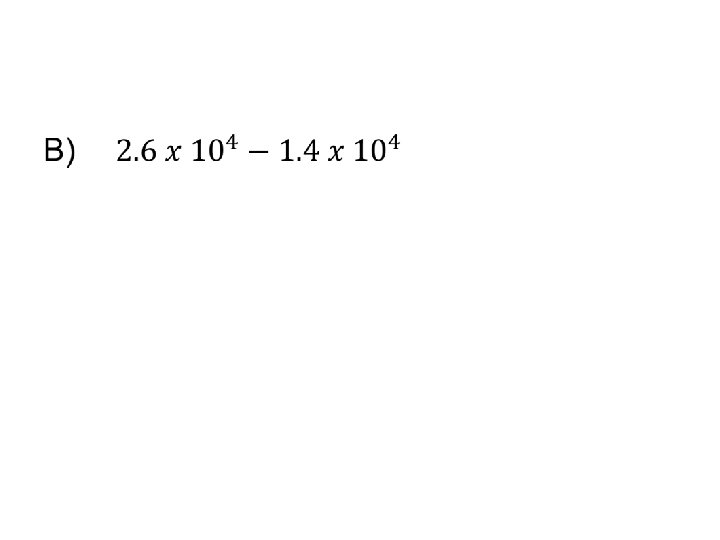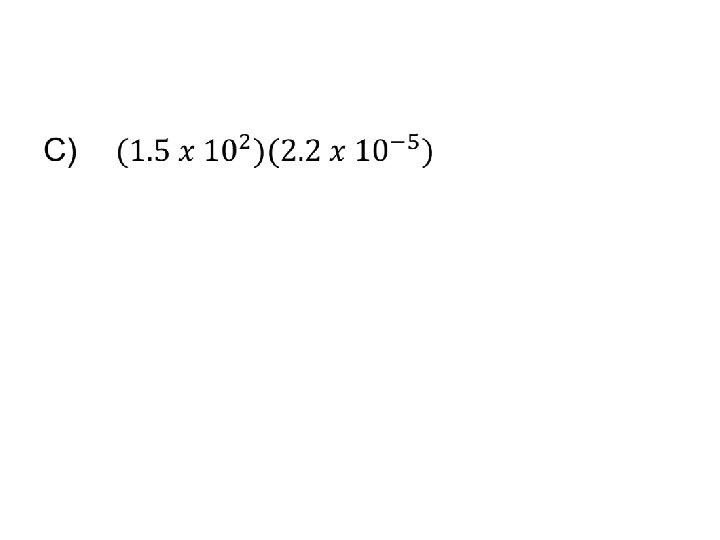# Physics Math Section 1 Scientific Notation Scientific Notation

• Slides: 47Physics MathSection 1: Scientific Notation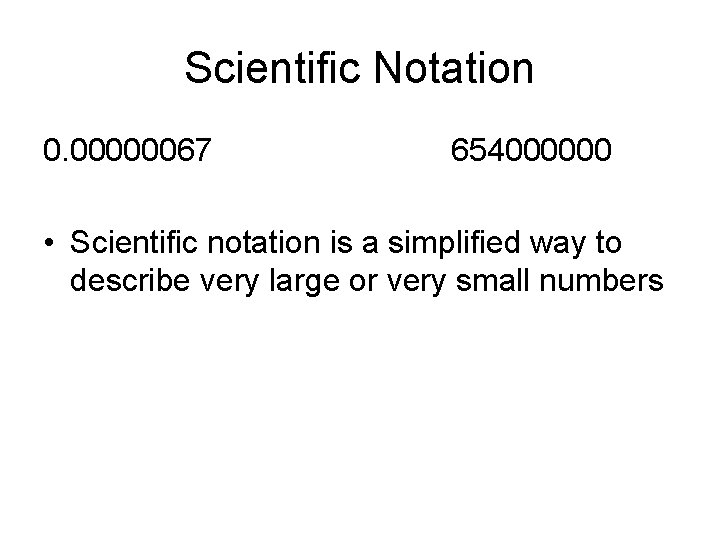Scientific Notation 0. 00000067 654000000 • Scientific notation is a simplified way to describe very large or very small numbers1. 2. 3. 4. 5. 6. 7. 8. 549000 0. 0000612 5680 0. 00356 3. 0 x 108 9. 0 x 109 6. 67 x 10 -11 3. 31 x 102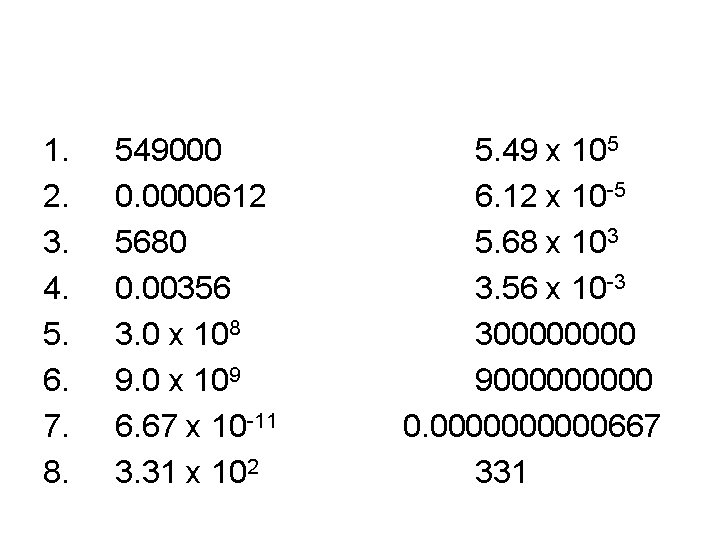1. 2. 3. 4. 5. 6. 7. 8. 549000 0. 0000612 5680 0. 00356 3. 0 x 108 9. 0 x 109 6. 67 x 10 -11 3. 31 x 102 5. 49 x 105 6. 12 x 10 -5 5. 68 x 103 3. 56 x 10 -3 30000 900000 0. 00000667 331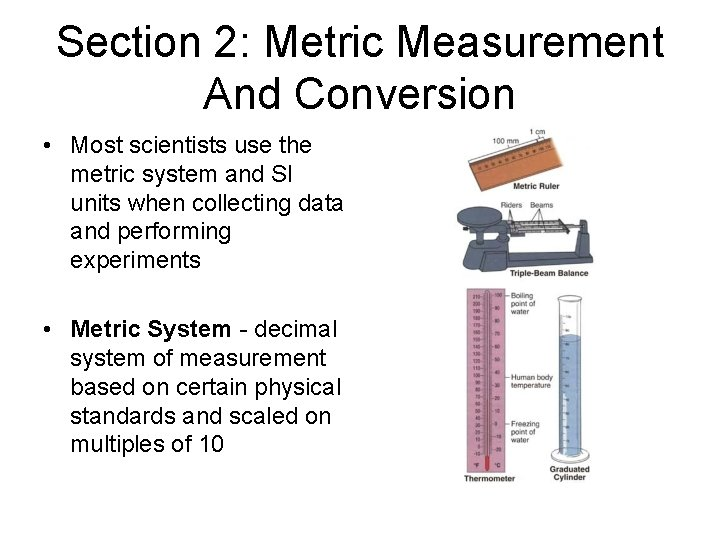Section 2: Metric Measurement And Conversion • Most scientists use the metric system and SI units when collecting data and performing experiments • Metric System - decimal system of measurement based on certain physical standards and scaled on multiples of 10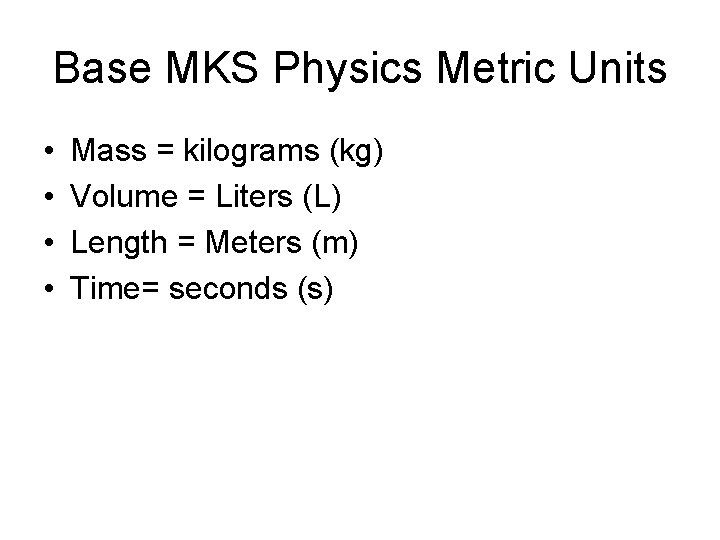Base MKS Physics Metric Units • • Mass = kilograms (kg) Volume = Liters (L) Length = Meters (m) Time= seconds (s)Metric Prefixes • A prefix describes the base unit either making the power of 10 times bigger or smallerCommon Bigger Than Base Prefixes Bigger than the base Smaller than the base If the base was meters Metric mega. Prefixes kilo- k hecto- deca- B deci- centi- milli- microa Kilometers (km) are s bigger than meters e h da d c m μ 1, 000 10 0. 1 1 1 M How 1, 000 many of the base unit 1000 meters in a 0. 01 0. 000001 kilometer How many of these smaller units in the base 10 unit 1 100 1000 1, 000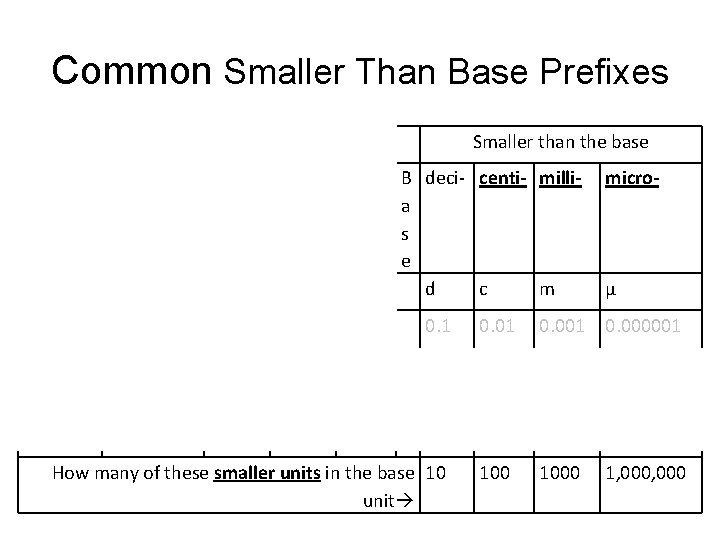Common Smaller Than Base Prefixes Bigger than the base Metric mega. Prefixes kilo- M How 1, 000 many of the base unit Smaller than the base micro- k hecto- deca- B deci- centi- millia s e h da d c m 1, 000 10 0. 1 0. 001 0. 000001 1 1 100 1000 1, 000 How many of these smaller units in the base 10 unit μBigger than the base Metric mega. Prefixes kilo- M How 1, 000 many of the base unit Smaller than the base micro- k hecto- deca- B deci- centi- millia s e h da d c m 1, 000 10 0. 1 1 How many of these smaller units in the base 10 unit μ 0. 01 0. 000001 1000 1, 000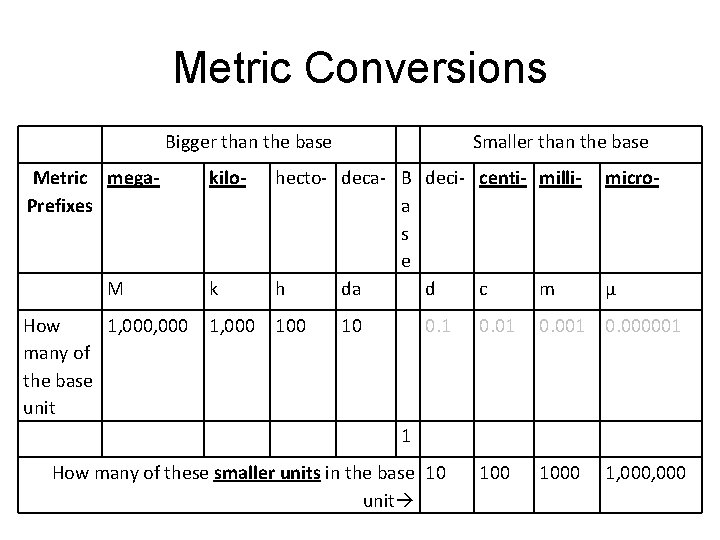Metric Conversions Bigger than the base Metric mega. Prefixes kilo- M How 1, 000 many of the base unit Smaller than the base micro- k hecto- deca- B deci- centi- millia s e h da d c m 1, 000 10 0. 1 1 How many of these smaller units in the base 10 unit μ 0. 01 0. 000001 1000 1, 000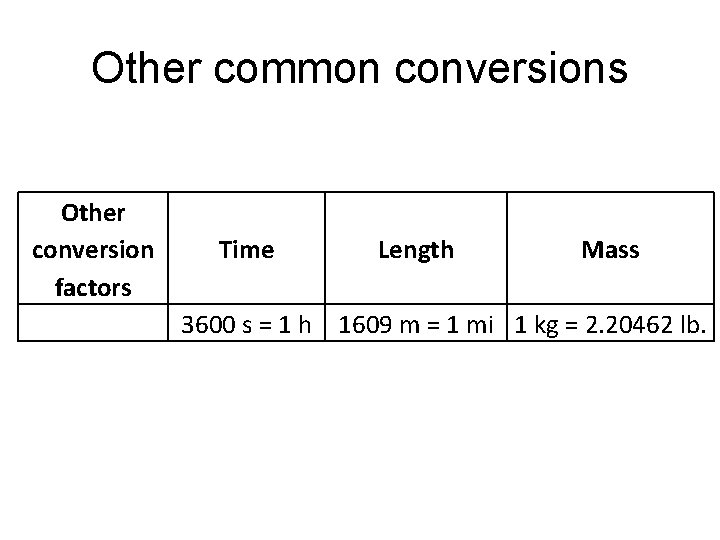Other common conversions Other conversion Time Length Mass factors 3600 s = 1 h 1609 m = 1 mi 1 kg = 2. 20462 lb.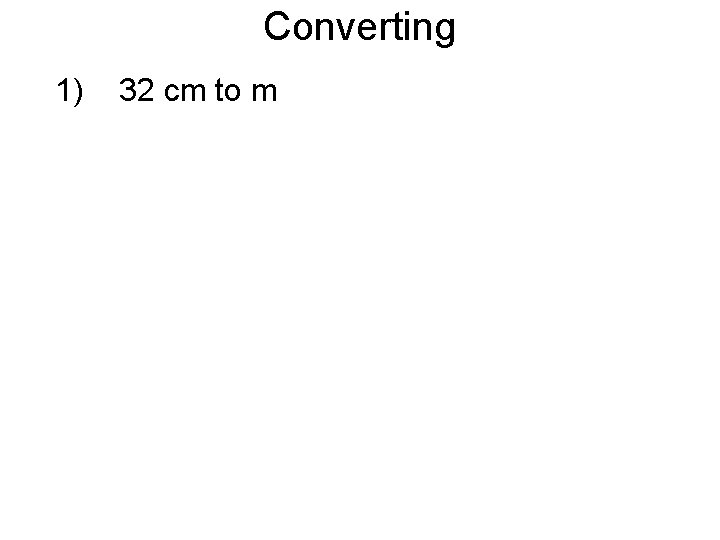Converting 1) 32 cm to m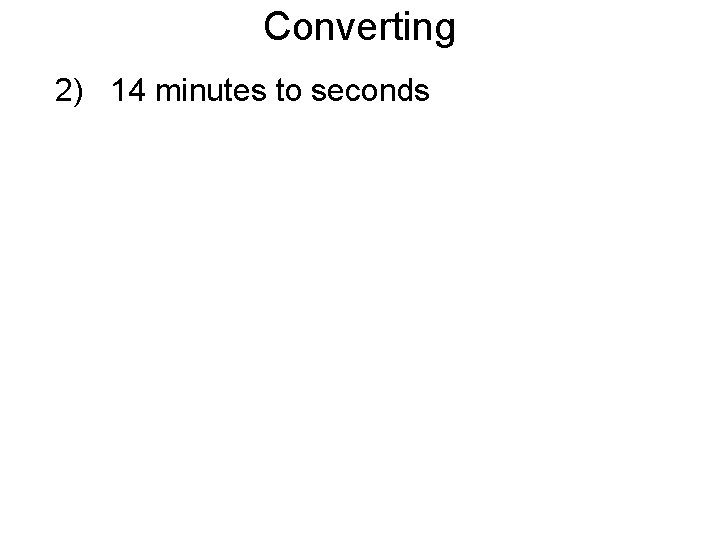Converting 2) 14 minutes to seconds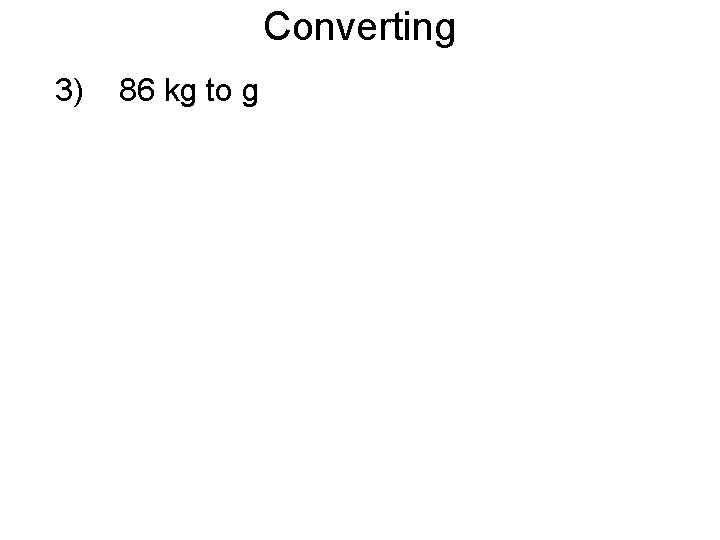Converting 3) 86 kg to g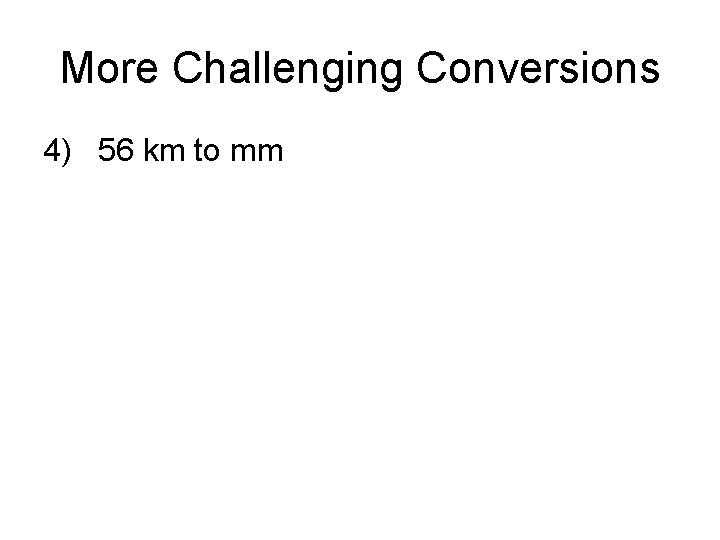More Challenging Conversions 4) 56 km to mm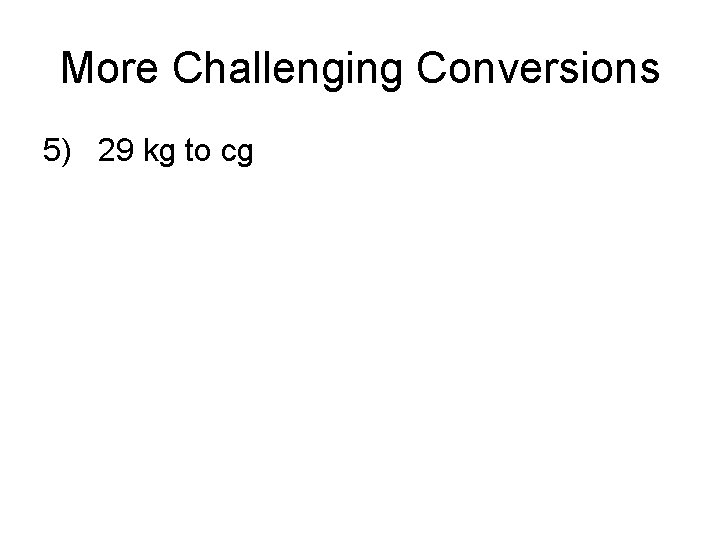More Challenging Conversions 5) 29 kg to cg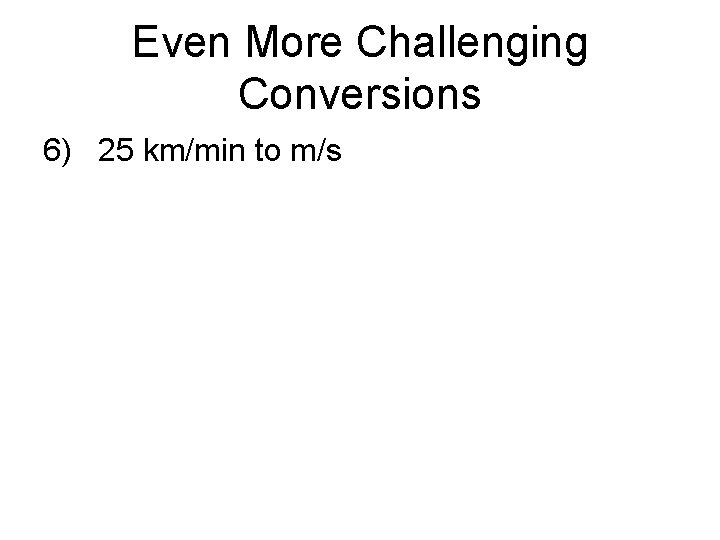Even More Challenging Conversions 6) 25 km/min to m/sSection 3: Physics Algebra1. Solve the equation above for x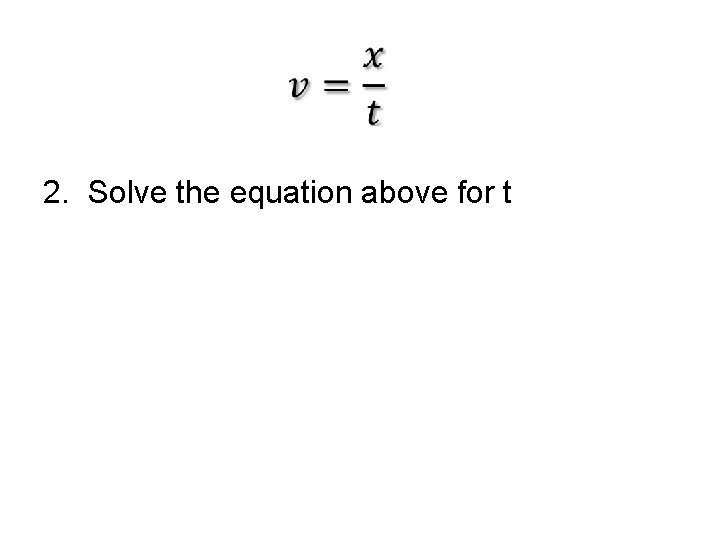2. Solve the equation above for t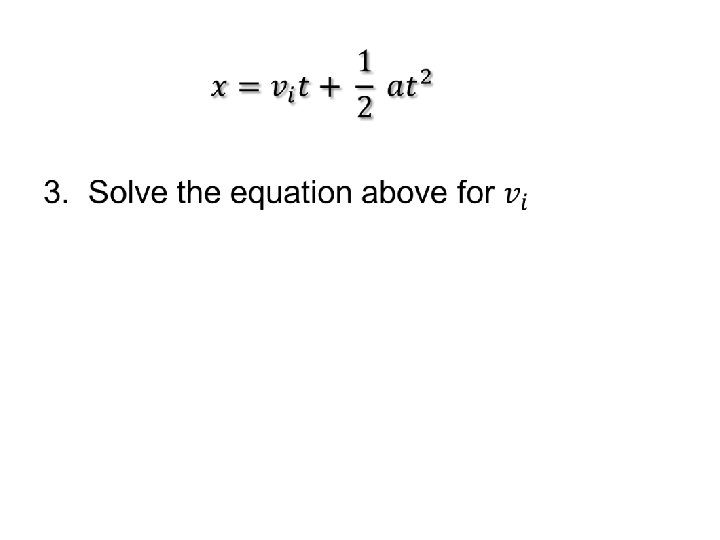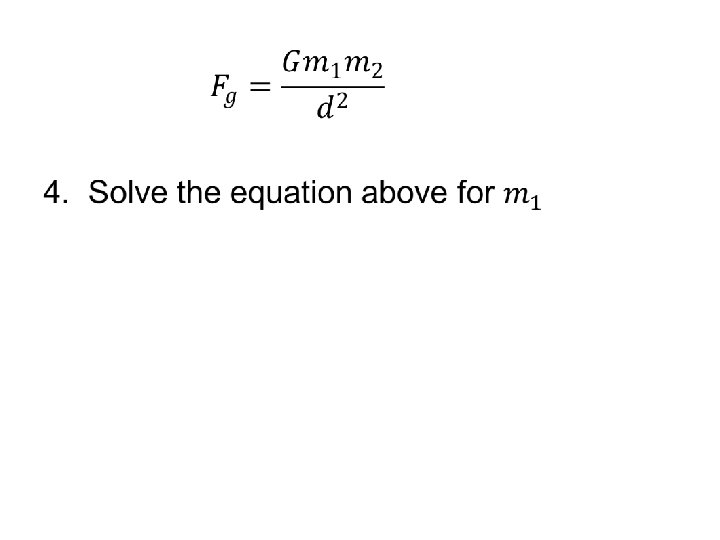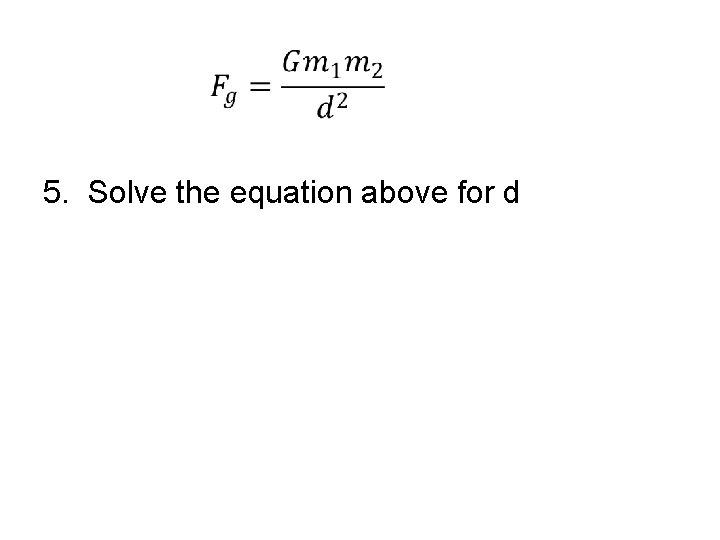5. Solve the equation above for d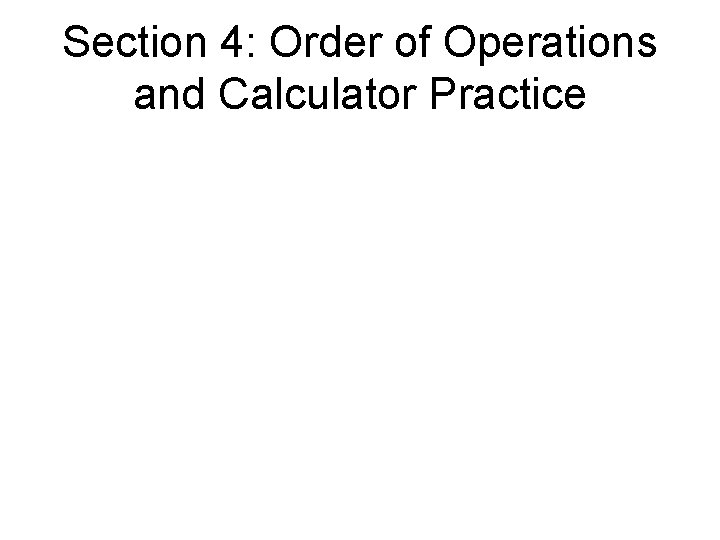Section 4: Order of Operations and Calculator PracticeOrder of Operations 10 - 5 ÷ (4 - 3) x 8 + 6 = Step 1: First perform any calculations inside parentheses. Step 2: Next perform all multiplications and divisions, working from left to right. Step 3: Lastly, perform all additions and subtractions, working from left to right.Order of Operations 10 - 5 ÷ 1 x 8 + 6 = Step 2: Next perform all multiplications and divisions, working from left to right.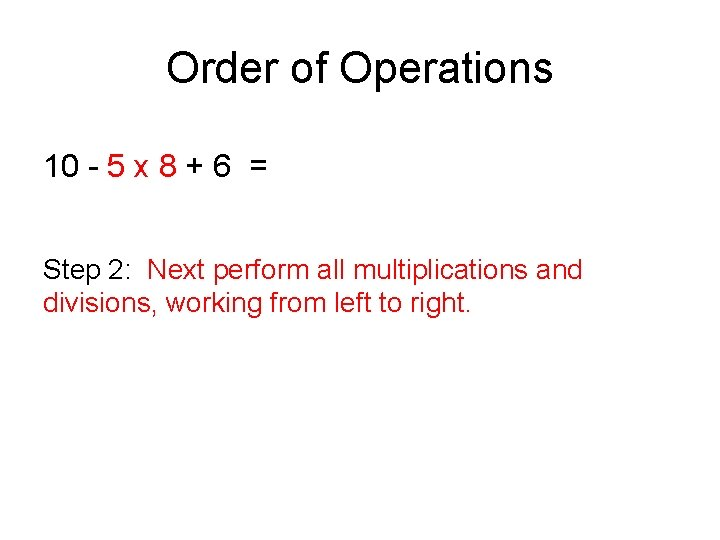Order of Operations 10 - 5 x 8 + 6 = Step 2: Next perform all multiplications and divisions, working from left to right.Order of Operations 10 - 40 + 6 = Step 3: Lastly, perform all additions and subtractions, working from left to right.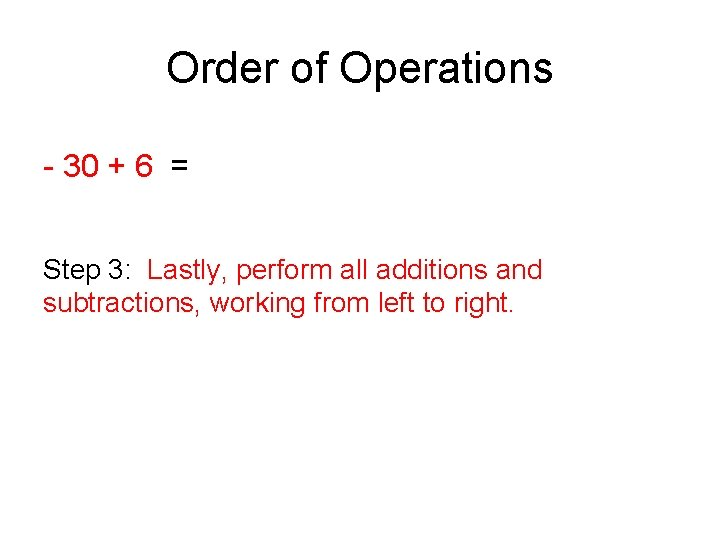Order of Operations - 30 + 6 = Step 3: Lastly, perform all additions and subtractions, working from left to right.Order of Operations: End Result 10 - 5 ÷ (4 - 3) x 8 + 6 = -24 Step 1: First perform any calculations inside parentheses. Step 2: Next perform all multiplications and divisions, working from left to right. Step 3: Lastly, perform all additions and subtractions, working from left to right.Scientific notation in a calculator ***Use parenthesis*** whenever you don’t know how your calculator will handle numbers You should not use carrots^ when using scientific notation 5 x 104 You would type instead 5 (exponent button) 4Scientific notation in a calculator 5 x 104 You would type instead 5 (exponent button) 4 Look for the exponent button which is different on various scientific calculatorsCommon Scientific Calculators EEEEEE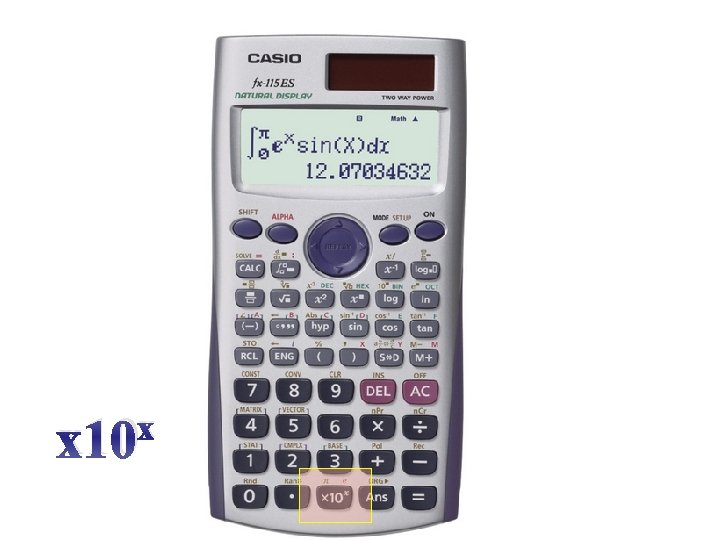x x 10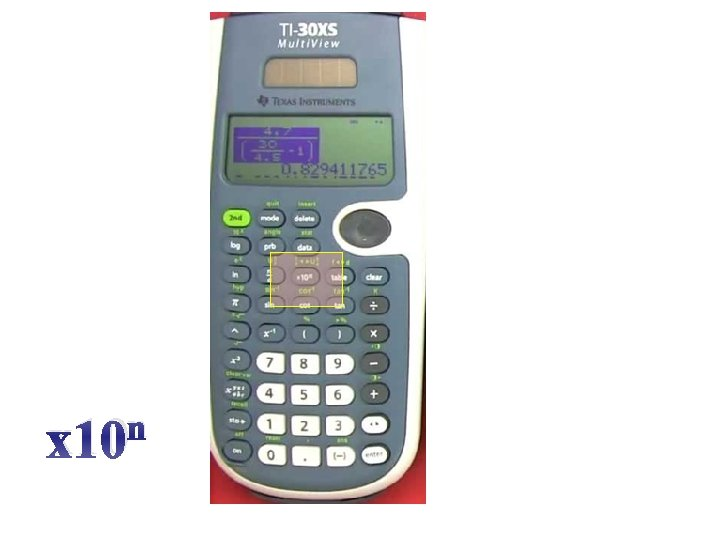n x 10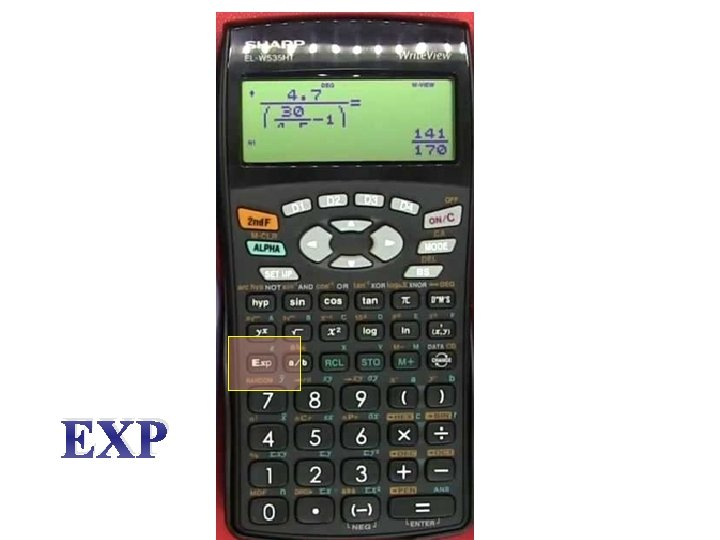EXP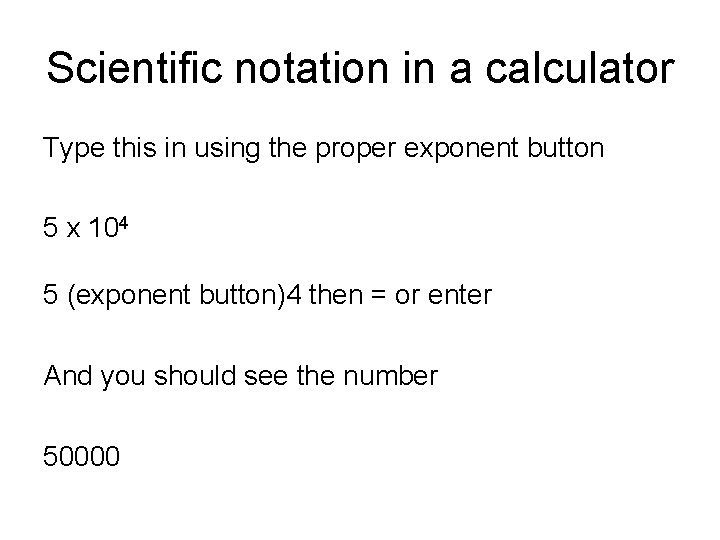Scientific notation in a calculator Type this in using the proper exponent button 5 x 104 5 (exponent button)4 then = or enter And you should see the number 50000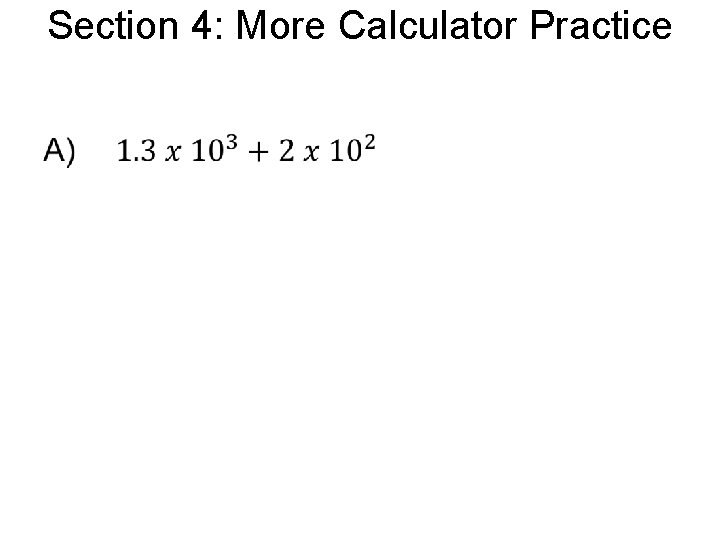Section 4: More Calculator Practice •